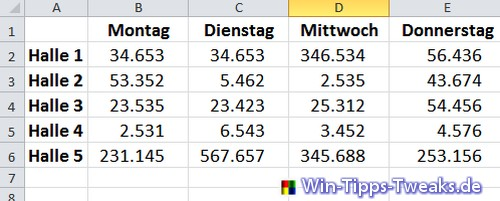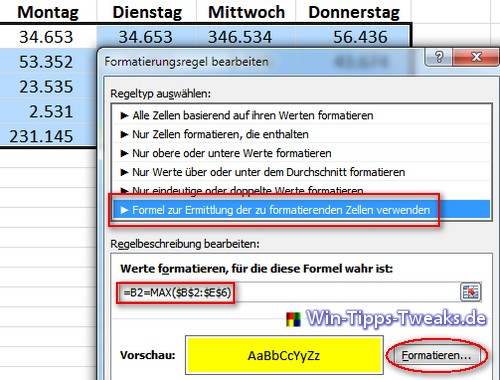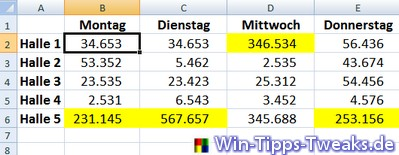# Excel 2010: Apply conditional formattingThe Excel function Conditional formatting should offer the possibility to format cells depending on certain conditions. There are different ones Rule typeswhich, when used correctly, make the formulas in Excel more productive. The time required for an evaluation can often be significantly reduced. The rule type is particularly interesting "Use formula to determine which cells to format". This enables meaningful evaluations to be created quickly.

Three Examples should show the possibilities once.

1. Mark the cell with the maximum value in a table

Here, the maximum value should be optically highlighted within any data record with the help of formatting. For this purpose, a table was created that shows the number of visitors to various exhibition halls on certain days.To begin with, let's start all of them Visitor numbers the table marked and the rider Home > Conditional formatting New Rule inserted.The first thing among the formatting rules is the rule type Use formula to determine which cells to format. The following formula is selected for the table above:

= B2 = MAX (\$ B \$ 2: \$ E \$ 6)Finally, click the button format, appropriate formatting selected for the cell to be selected. Here was chosen for a yellow filling .2. Mark the maximum value of each line

The maximum value of each line, i.e. each hall, should be marked here. So there will be five values. Since the Procedure identical like at first Example only the formula is adjusted.

= B2 = MAX (\$ B2: \$ E2)3. Mark the maximum value of each column

The maximum value of each column, i.e. each day, should be marked here. It will be four values. Here, too, only the formula is adjusted.

= B2 = MAX (B \$ 2: B \$ 6)___________________________________________________
This tip comes from www.win-tipps-tweaks.de